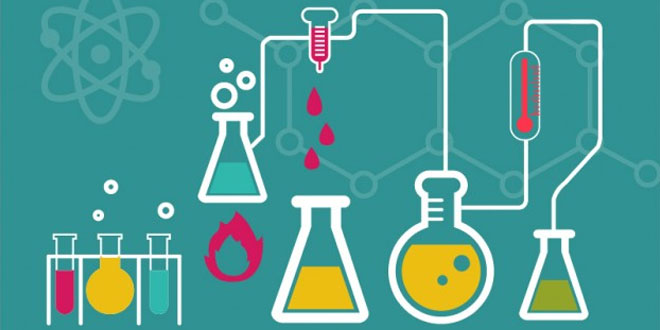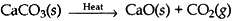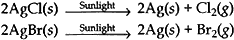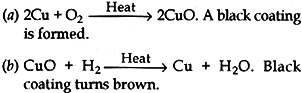Wednesday , May 18 2022# Chemical Reaction & Equations: 10 Science

#### Question: What type of coating is formed on silver articles when they get corroded?

Answer: The silver articles form black coating of silver sulphide (Ag2S).

#### Question: What type of a coating is formed on copper articles when they get corroded?

Answer: The copper articles form green coating of basic copper carbonate [CuCO3.Cu(OH)2]

#### Question: Balance the following chemical equation: Fe(s)+H2O(g) → Fe3O4(s) + H2(g)

Answer: 3Fe(s)+4H2O(g) → Fe3O4(s) + 4H2(g)

#### Question: What is meant by skeletal equation?

Answer: Unbalanced chemical is called a skeletal equation.

#### Question: If copper metal is heated over a flame, it develops a coating. What is the colour and composition of this coating?

CuO or Copper oxide

#### Question: All decomposition reactions are endothermic reactions. Explain why.

Answer: Because energy in the form of heat, light or electricity is required for decomposition reactions.

#### Question: Sate what change in the colour of iron nail and copper sulphate solution are observed when an iron nail is dipped in copper sulphate solution for about 20 minutes. Write the equation for the reaction that takes place. Also, name the type of reaction involved in the above process.

1. The iron nail becomes brownish and the blue colour of copper sulphate solution fades.
2. Fe(s) + CuSO4(aq) → FeSO4(aq) + Cu(s)
3. Displacement reaction.

#### Question: Can oxidation or reduction take alone? Why or why not? What are such reactions called?

Answer: No. In a chemical reaction, if one substance is oxidized, then other will be reduced such reactions are called redox reaction.

#### Question: Explain giving chemical equation any two uses of chemical decomposition reaction in industry.

Answer: (i) In manufacturing cement, quicklime is used and it is obtained by thermal decomposition of limestone.(ii) In manufacturing photochromic glass, silver chloride/bromide is used which turns grey in sunlight.#### (1) Vegetable matter changing into compost. (2) Burning of natural gas. (3) Silver items turning black when left exposed ti air. (4) Change in colour of copper sulphate for blue and green on dipping a nail in it.

1. Decomposition reaction
2. Oxidation
3. Oxidation reaction
4. Displacement reaction

#### (ii) Mention the inference you draw regarding the reactivity of metal ‘X’ and ‘Y’ and also about the type of reaction. State the reason of your conclusions.

Answer: It is a displacement reaction.
(i) Mental X is more reactive than metal Y.
(ii) Mental X is displacing metal Y from its salt solution. A more reactive metal displaces less reactive one from its salt solution, C is more reactive than Y.

#### Question: Giving chemical equation answer the following: (a) What happens when copper is heated in air? (b) What happens when the product obtained in above reaction is heated in hydrogen?